# Analytic surface (in algebraic geometry)

A two-dimensional (complex) analytic manifold, i.e. a smooth four-dimensional manifold with a complex structure. While the theory of analytic surfaces forms part of the general theory of complex manifolds, the two-dimensional case is treated separately, since much more is known about analytic surfaces than about-dimensional manifolds if. Moreover, certain facts are specific to the two-dimensional case alone. These results concern the classification of analytic surfaces, which is analogous to that of algebraic surfaces (cf. Algebraic surface) — a fact which largely reduces the theory of analytic surfaces to that of algebraic surfaces. The principal results on the classification of analytic surfaces were obtained by K. Kodaira , , , but his work is based on the results of the classical Italian school of algebraic geometry on the classification of algebraic surfaces.

All analytic surfaces discussed below are assumed to be compact and connected.

## Contents

### Examples.

1) Algebraic surfaces. Let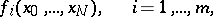be a set of homogeneous polynomials with complex coefficients. The closed subset of the complex projective space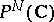specified by the equationsis an analytic surface if it is non-singular, connected and has complex dimension two. This is the basic example of an analytic surface.

2) Complex tori. Letbe the two-dimensional vector space over the field of complex numbers (as a vector space over the field of real numbers it is isomorphic to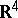) and letbe a lattice in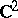. The quotient spaceis an analytic surface. Being a smooth manifold,is diffeomorphic to a four-dimensional torus, but the complex structure ondepends on the lattice. Complex toriplay an important role in analysis, since meromorphic functions on such tori are meromorphic functions onand are periodic with period lattice. Analytic surfaces of the type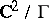are not always algebraic. There also exist latticessuch that there are no meromorphic functions at all (except for constants) on the corresponding torus. For specific examples of such tori see .

3) Hopf surfaces. Let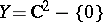and letbe a positive number. Consider the action of the group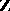on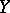given by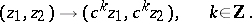The groupacts discretely and without fixed points on, while the quotient spaceis diffeomorphic to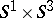. The quotient spacehas a natural structure of an analytic surface, and is called a Hopf surface.

## Classification of analytic surfaces.

The principal invariant in the classification of analytic surfaces is the transcendence degree of the field of meromorphic functionson the analytic surface. According to Siegel's theorem, for any compact connected manifoldthe field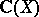is finitely generated, and its transcendence degree is not larger than the complex dimension of. Thus, for an analytic surface, the fieldcontains two independent meromorphic algebraic functions, or one such function or constants only. These possibilities lead to the following theorems.

For any analytic surfaceto be an algebraic surface it is necessary and sufficient that there exist two algebraically independent meromorphic functions on.

If an analytic surfacehas a field of meromorphic functions of transcendence degree 1, thenis an elliptic surface, i.e. there is a holomorphic mapping onto an algebraic curve,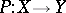, such thatand all fibres of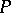, except for a finite number, are elliptic curves (the singular fibres may only have a very special form, which has been thoroughly studied).

If no meromorphic functions other than constants exist on an analytic surface, and no exceptional curves (cf. Exceptional subvariety) exist on, then the first Betti numberofassumes only three values: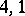or 0. If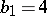,is a complex torus, and if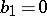,has a trivial canonical fibration. These analytic surfaces are called-surfaces. They are all mutually homeomorphic. The casehas not been studied in detail, but certain examples of analytic surfaces withare obtained by a generalization of the construction of Hopf surfaces.

Analytic Kähler surfaces are not always algebraic. They are, however, algebraic if the square of their first Chern class is positive. All analytic Kähler surfaces with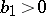are deformations of algebraic surfaces.